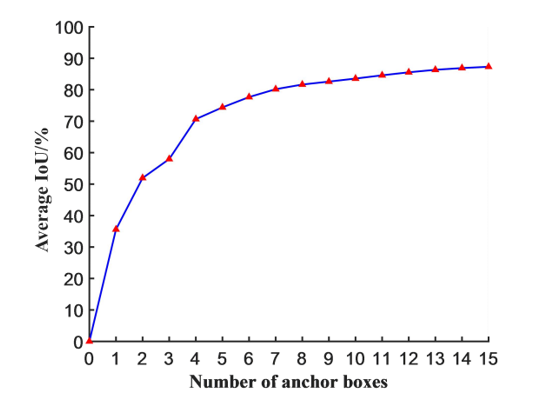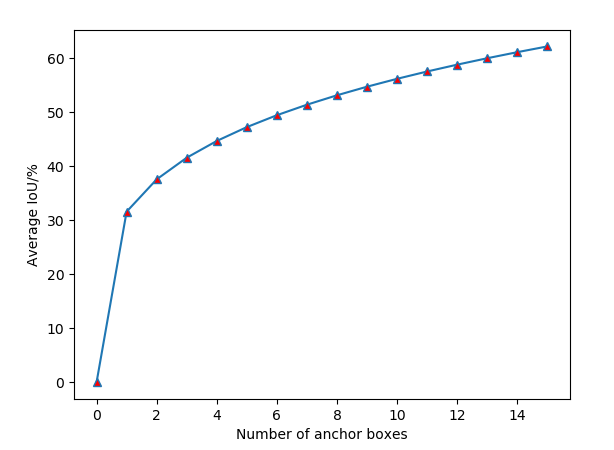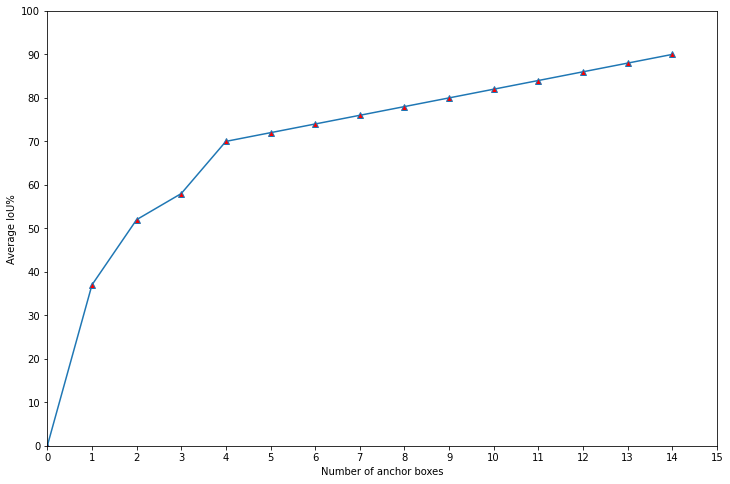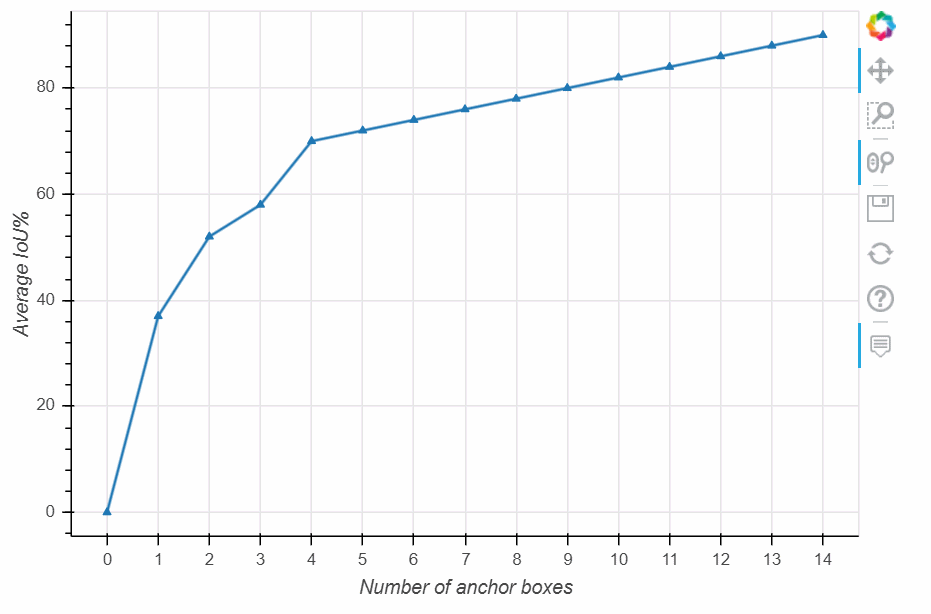2021-04-02 21:51

# Python怎样画折线图• 写回答
• 好问题 提建议
• 关注问题
• 收藏
• 邀请回答

#### 4条回答默认 最新

•AI 菌 2021-04-02 22:49
已采纳

根据你的问题，下面专门为你解答，可直接运行下面代码：

``````import matplotlib.pyplot as plt

# 设置图框的大小
fig = plt.figure(figsize=(10,6))

x = [0,1, 2, 3,4,5,6,7,8,9,10]
y = [0,25,40,50,58,65,71,76,80,82,88]

# 绘图
plt.plot(x, # x轴数据
y, # y轴数据
linestyle = '-', # 折线类型
linewidth = 2, # 折线宽度
color = 'steelblue', # 折线颜色
marker = '*', # 点的形状
markersize = 6, # 点的大小
markeredgecolor='black', # 点的边框色
markerfacecolor='green') # 点的填充色

# 添加标题和坐标轴标签
plt.xlabel('Number of anchor boxes')
plt.ylabel('Average IoU/%')
plt.show()``````
已采纳该答案
评论
解决 无用
打赏 举报
•cc__cc__ 2021-04-02 22:11
评论
解决 无用
打赏 举报
•天元浪子 2021-04-02 22:59

试试这个代码。

``````>>> import numpy as np
>>> import matplotlib.pyplot as plt
>>> x = np.arange(16)
>>> y = np.power(x/100, 1/4) * 100
>>> plt.plot(x, y, marker='^', mfc='red')
[<matplotlib.lines.Line2D object at 0x0000026174ED0CC8>]
>>> plt.xlabel('Number of anchor boxes')
Text(0.5, 0, 'Number of anchor boxes')
>>> plt.ylabel('Average IoU/%')
Text(0, 0.5, 'Average IoU/%')
>>> plt.show()``````评论
解决 无用
打赏 举报
•提供两种方法, 一种用pandas 一种用pandas-bokeh

``````# 方法一
import numpy as np
import pandas as pd

data = pd.DataFrame([0,37,52,58,70,72,74,76,78,80,82,84,86,88,90],columns=['值'])

data.plot(figsize=(12,8), # 图表大小
xlim=(0,15),  # x轴范围
ylim=(0,100), # y轴范围
yticks=range(0,110,10), #y轴间隔
xticks=range(0,16,1),  # x轴间隔
xlabel='Number of anchor boxes', #x轴名称
ylabel='Average IoU%',  # y轴名称
marker='^',  # 标记
legend=None, # 不显示图例
mfc='red',  #标记颜色
)

# 方法二
import numpy as np
import pandas as pd
import pandas_bokeh

data = pd.DataFrame([0,37,52,58,70,72,74,76,78,80,82,84,86,88,90],columns=['值'])

data[['值']].plot_bokeh(xlabel='Number of anchor boxes',
ylabel='Average IoU%',
plot_data_points=True,
legend=None, # 不显示图例
marker='^',  # 标记
)``````可参考:

https://blog.csdn.net/muyashui/category_10867984.html

评论
解决 无用
打赏 举报